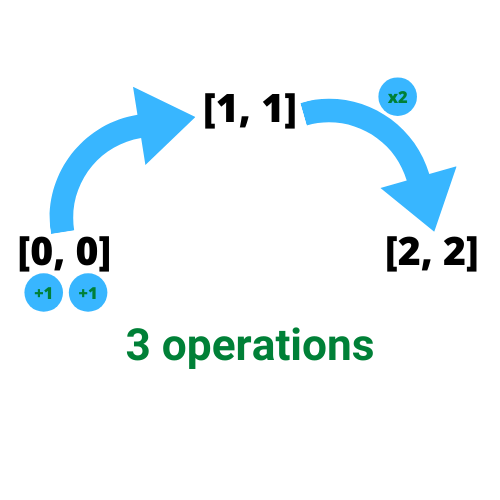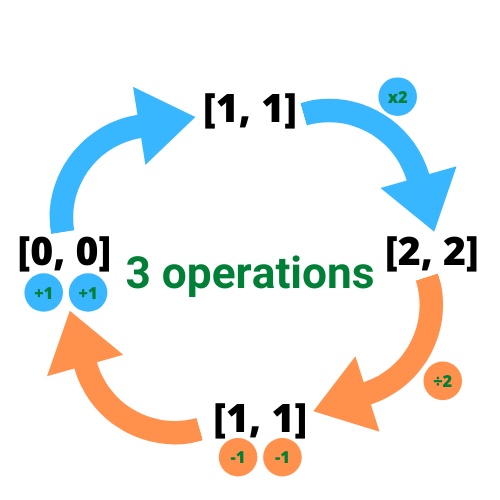# Minimum Numbers of Function Calls to Make Target Array With Python and Javascript

Created on Oct 21, 2020
Updated on Oct 22, 2020

## Understanding the Problem

Minimum Numbers of Function Calls to Make Target Array is a medium problem at leetcode, I hope you have read the problem carefully and have tried solving it. This problem requires you to get the least number of operations that you can do in order to get to the desired array. The question now, how we can do such operations to count its numbers to reach the target array in the least possible way.

## Initial thoughts

Let us take this example, having [2, 2] as the target array and we want to know the minimum number of operations to reach that array. So we start off with [0, 0] adding 1 to each element (2 operations now) reaching [1, 1] and then multiply the whole array by 2 (1 operation) reaching the target array we want [2, 2] so we have 3 operations to reach the desired array for this example as shown below:Image by the Author

But it’s hard to move from [0, 0] to reach [2, 2] because of the possibility of wrong guessing of the next array we want to reach. What we can better do, is to move backward from [2, 2] to [0, 0] as indicated below:Image by the Author

In this case, we can divide instead of multiplying and subtracting instead of adding as shown in orange and we’ll end up with the same number of operations.

## Better path

So now we have two main operations to be done: division and subtraction. It seems that we use division when all elements in the array are divisible by 2 but what if there is an odd number, we subtract. The integer division can save us from worrying about the existence of odd numbers because even and odd numbers will be divided and what’s left is the count of operations for every odd number existing which can be done by calculating the remainder. The remainder of any number by 2 is either 1 or 0. If 1, we indicate that the number is odd and then we know that this is one operation.

Here are some scripts of how that integer division and the remainder are calculated in Python and Javascript:

## Acknowledgment

The Python code is a mutual work with my best friend Nour . He actually has a contribution at gist for explaining a more efficient solution found on leetcode, please see this fantastic gist:

## More problem-solving?

If you like this, you might want to take a look at:

If you haven’t followed me on medium , please do so. Thank you! :)